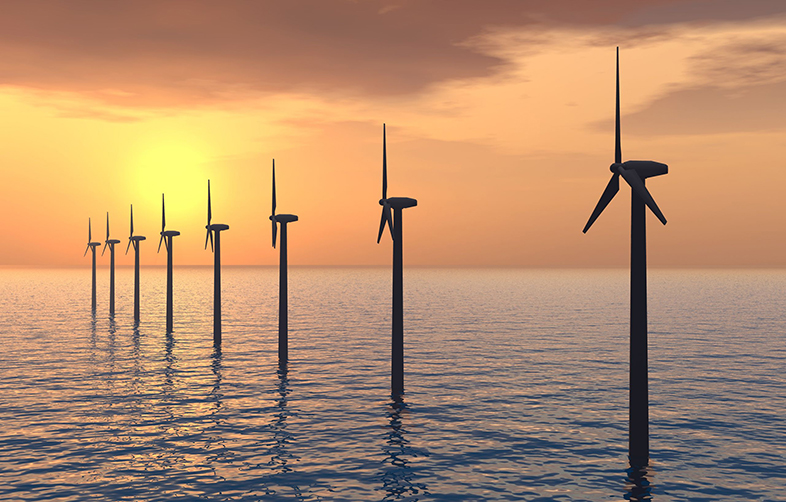Can renewable energy sources power the world?

Start this free course now. Just create an account and sign in. Enrol and complete the course for a free statement of participation or digital badge if available.

Free course

# 2.1 Units of energy and power

A host of different units are used to describe energy and power, which can seem a bit confusing. But the following Table 1, where we have listed and described some of the most frequently used units, should clarify the meaning of the various terms.

## Table 1 Common units of energy and power

EnergyPower
NameDescriptionNameDescription
Joule (J)Main scientific unit of energyWatt (W)Main scientific unit of power – defined as 1 joule per second
Kilojoule (KJ)Equal to 1000 (103) joulesMilliwatt (mW)Equal to 1000th of a watt (10-3)
Megajoule (MJ)Equal to 1 million (106) joulesKilowatt (kW)Equal to 1000 (103) watts
Gigajoule (GJ)Equal to 1 billion (109) jouleMegawatt (MW)Equal to 1 million (106) watts
Exajoule (EJ)Equal to 1 quintillion (1018) joulesGigawatt (GW)Equal to 1 billion (109) watts
Kilowatt-hour (kWh)The amount of energy produced by a power of 1 Kilowatt (1 kW) in one hour
Megawatt-hour (MWh)The amount of energy produced by a power of 1 Megawatt (1 MW) in one hour
Gigawatt-hour (GWh)The amount of energy produced by a power of 1 Gigawatt (1 GW) in one hour

As you can see from Table 1, most of the terms are used to describe larger quantities of the basic units – joules or watts.

RENBOC_1